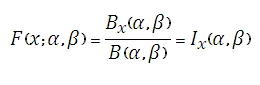# SQL Server BETA.DIST Function

Updated: 9 August 2010

Use BETADIST to calculate the beta cumulative probability density function.Where
Bx(α,β) is the incomplete beta function and
Ix(α,β) is the regularized incomplete beta function.
Syntax
SELECT [wctStatistics].[wct].[BETADIST] (
<@X, float,>
,<@P, float,>
,<@Q, float,>
,<@A, float,>
,<@B, float,>)
Arguments
@X
is any real number. @X is an expression of type float or of a type that can be implicitly converted to float@X is the value between @P and @Q at which to evaluate the function
@P
is any real number. @P is an expression of type float or of a type that can be implicitly converted to float@A is a parameter to the distribution
@Q
is any real number. @Q is an expression of type float or of a type that can be implicitly converted to float@Q is a parameter to the distribution
@A
is any real number. @A is an expression of type float or of a type that can be implicitly converted to float@A is the lower bound to the distribution. If you enter NULL, @A defaults to zero
@B
is any real number. @B is an expression of type float or of a type that can be implicitly converted to float.   @B is the upper bound to the distribution. If you enter NULL @B defaults to to zero.

Return Types
float
Remarks
·         If @X ≤ 0, BETADIST will return an error.
·         If @P ≤ 0 or @Q ≤ 0, BETADIST will return an error
·         If @X< @A or @X > @B or @A = @B, BETADIST will return an error.
·         If you enter NULL for @A and @B, BETADIST will use zero and one.
Examples
select wct.BETADIST(7,5,10,6,8)

This produces the following result

----------------------
0.91021728515625

(1 row(s) affected)

### PricingCopyright 2008-2021 Westclintech LLC         Privacy Policy        Terms of Service